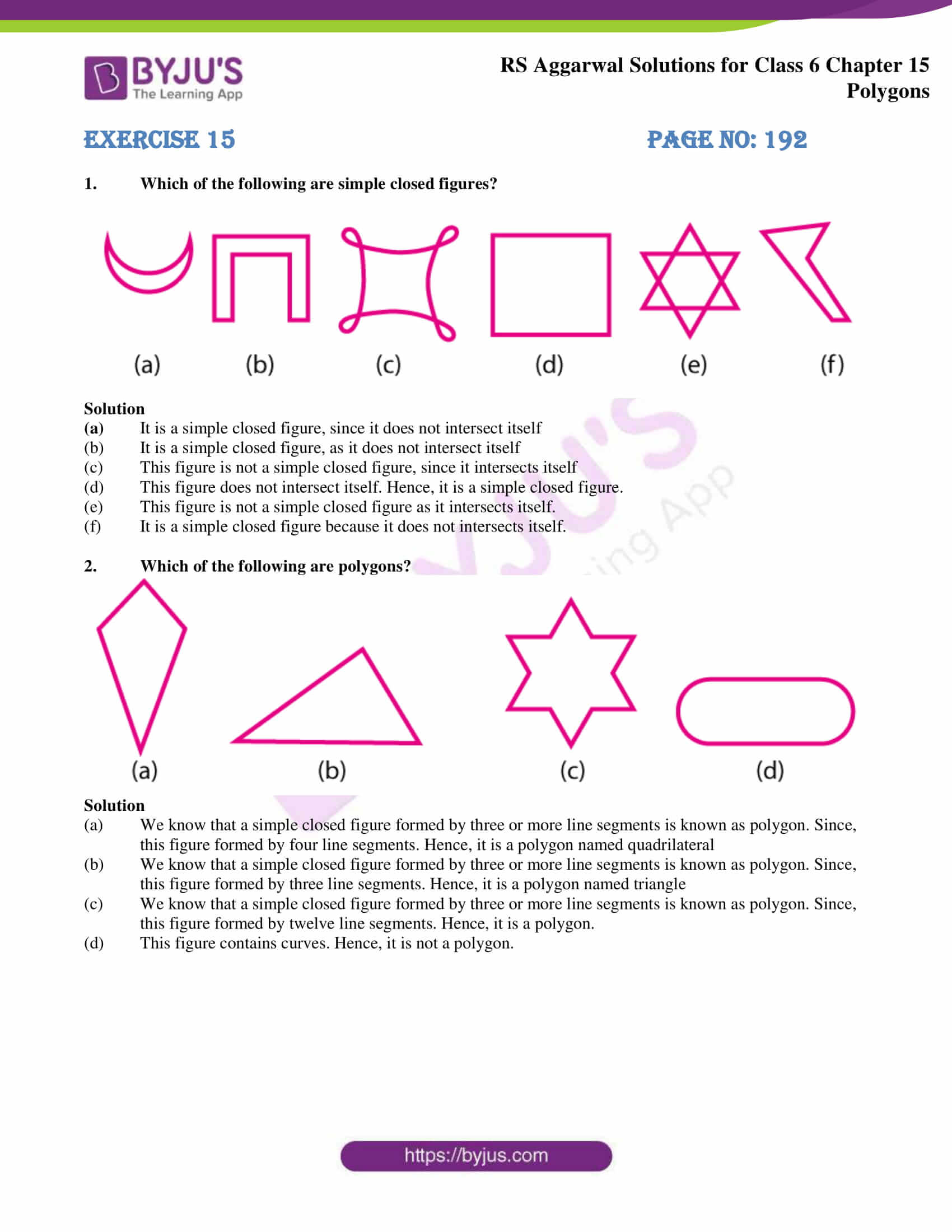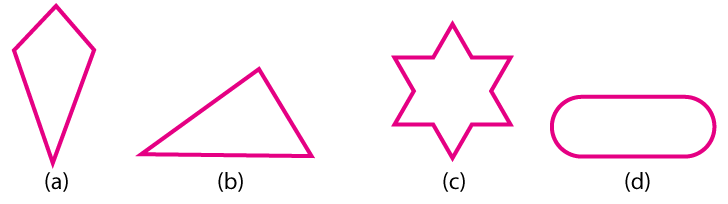# RS Aggarwal Solutions for Class 6 Maths Chapter 15 Polygons

RS Aggarwal Solutions for Class 6 Chapter 15 are provided here for students in PDF format. Students who practice RS Aggarwal Solutions will excel in examination with good marks along with gaining confidence in Mathematics. Practicing RS Aggarwal textbook regularly will help students in clearing their doubts related to the chapter.

Experts in Mathematics at BYJU’S solved RS Aggarwal textbook questions for Class 6 Chapter 15 in simple PDF format. Download PDF of Class 6 Chapter 15 in their respective links.

## Download PDF of RS Aggarwal Solutions for Class 6 Chapter 15 Polygons### Access answers to chapter 15 – Polygons

Exercise 15 PAGE No: 192

1. Which of the following are simple closed figures?Solution

(a) It is a simple closed figure, since it does not intersect itself

(b) It is a simple closed figure, as it does not intersect itself

(c) This figure is not a simple closed figure, since it intersects itself

(d) This figure does not intersect itself. Hence, it is a simple closed figure.

(e) This figure is not a simple closed figure as it intersects itself.

(f) It is a simple closed figure because it does not intersects itself.

2. Which of the following are polygons?Solution

(a) We know that a simple closed figure formed by three or more line segments is known as polygon. Since, this figure formed by four line segments. Hence, it is a polygon named quadrilateral

(b) We know that a simple closed figure formed by three or more line segments is known as polygon. Since, this figure formed by three line segments. Hence, it is a polygon named triangle

(c) We know that a simple closed figure formed by three or more line segments is known as polygon. Since, this figure formed by twelve line segments. Hence, it is a polygon.

(d) This figure contains curves. Hence, it is not a polygon.

### RS Aggarwal Solutions for Class 6 Chapter 15 Polygons

Chapter 15 – Polygons has 1 exercise. Each question of this exercise is given with thorough explanations about Polygons. Let’s have a look at the topics mentioned in Polygons.

• Straight lines
• Curve
• Closed figures
• Open figures
• Simple closed figures
• Polygons
• Sides
• Vertices
• Diagonals
• Triangle

Also, access RS Aggarwal Solutions for Class 6 Chapter 15 Exercise

### Chapter Brief of RS Aggarwal Solutions for Class 6 Chapter 15 Polygons

Any two dimensional shape that is formed with straight lines is called a Polygon. The list of polygon name, depending on the number of sides they have are

Triangle – 3 sides

Pentagon – 5 sides

Hexagon – 6 sides

Heptagon – 7 sides

Octagon – 8 sides

There are two types of polygons

Regular polygons which have equal angles and sides

Irregular polygons which do not have equal angles and sides. Chairs, television, tiles are examples of polygons used in daily life.